# 9.1 Implementation

This section describes the technical details of the ArtiSynth skinning mechanism. A skin mesh is implemented using a SkinMeshBody, which contains a base mesh and references to a set of underlying dynamic master bodies. A master body can be either a Frame (of which RigidBody is a subclass), or a FemModel3d. The positions of the mesh vertices (along with markers and other points that can be attached to the skin mesh) are determined by a weighted sum of influences from each of the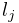master bodies, such that as the latter move and/or deform, the vertices and attached points deform as well. More precisely, for each master body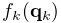, let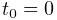be the weighting factor and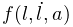the connection function that describes the contribution of body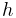to the position of the vertices (or attached points) as a function of its generalized coordinates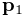. Then if the position of a vertex (or attached point) is denoted by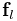and its initial (or base) position is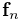, we have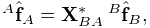(9.1)

The weight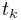in the last term is known as the base weight and describes an optional contribution from the base position. Usually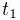, unless the vertex is not connected to any master bodies, in which case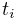, so that the vertex is anchored to its initial position.

In general, connection weightsare computed based on the distances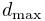between the vertex (or attached point) and each master body. More details on this are given in Sections 9.2 and 9.3.

For Frame master bodies, the connection function is one associated with various rigid body skinning techniques known in the literature. These include linear, linear dual quaternion, and iterative dual quaternion skinning. Which technique is used is determined by the frameBlending property of the SkinMeshBody, which can be queried or set in code using the methods

FrameBlending getFrameBlending()
void setFrameBlending (FrameBlending blending)

where FrameBlending is an enumerated type defined by SkinMeshBody with the following values:

LINEAR

Linear blending, in which the connection function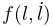implements a standard rigid connection between the vertex and the frame coordinates. Let the frame’s generalized coordinatesbe given by the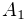rotation matrix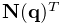and translation vector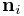describing its pose, with its initial pose given by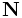and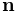. The connection functionthen takes the form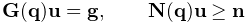(9.2)

Linear blending is faster than other blending techniques but is more prone to pinching and creasing artifacts in the presence of large rotations between frames.

DUAL_QUATERNION_LINEAR

Linear dual quaternion blending, which is more computationally expensive but typically gives better results than linear blending, and is described in detail as DLB in . Let the frame’s generalized coordinatesbe given by the dual-quaternion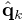(describing both rotation and translation), with the initial pose given by the dual-quaternion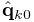. Then define the relative dual-quaternionas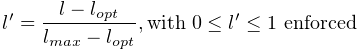(9.3)

where the denominator is formed by summing over all master bodies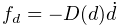which are frames. The connection functionis then given by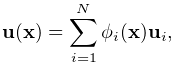(9.4)

where we note that a dual quaternion multiplied by a position vector yields a position vector.

DUAL_QUATERNION_ITERATIVE

Dual quaternion iterative blending, which is a more complex dual quaternion technique described in detail as DIB in . The connection function for iterative dual quaternion blending involves an iterative process and is not described here. It also does not conform to (9.1), because the connection functionsfor the Frame master bodies do not combine linearly. Instead, if there are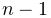Frame master bodies, there is a single connection function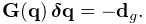(9.5)

that determines the connection for all of them, given their weighting factors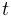and generalized coordinates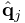. Iterative blending relies on two parameters: a blend tolerance, and a maximum number of blend steps, both of which are controlled by the SkinMeshBody properties DQBlendTolerance and DQMaxBlendSteps, which have default values of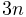and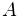.

Iterative dual quaternion blending is not completely supported in ArtiSynth. In particular, because of its complexity, the associated force and velocity mappings are computed using the simpler computations employed for linear dual quaternion blending. For the examples shown in this chapter, iterative dual quaternion gives results that are quite close to those of linear dual quaternion blending.

For FEM master bodies, the connection works by tying each vertex (or attached point) to a specific FEM element using a fixed-length offset vector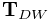that rotates in conjunction with the element. This is illustrated in Figure 9.2 for the case of a single FEM master body. Starting with the initial vertex position, we find the nearest point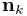on the nearest FEM element, along with the offset vector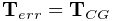. The pointcan be expressed as the weighted sum of the initial element nodal positions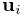,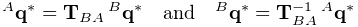(9.6)

where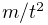is the number of nodes and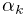represent the (constant) nodal coordinates. As the element moves and deforms, the element point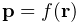moves with the nodal positions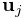according to the same relationship, while the offset vectorrotates according to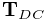, where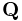is the rotation of the element’s coordinate frame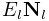with respect to its initial orientation. The connection functionthen takes the form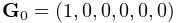(9.7)is determined by computing a polar decomposition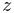on the deformation gradient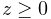at the element’s center. We note that the displacementis only rotated and so the distance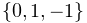of the vertex from the element remains constant. If the vertex is initially on or inside the element, then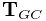and (9.7) takes the form of a standard point/element attachment as described in 6.4.3.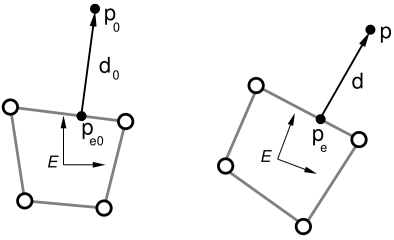Figure 9.2: Illustration of FEM skinning, showing how a positionis tied to an FEM element. Given the initial position, we find the nearest pointon the element, along with the offset vector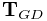(left). As the element moves and deforms, the updated position is obtained from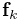, wheredeforms with the element, androtates in tandem with its coordinate frame.

While it is sometimes possible to determine weightsthat control a vertex position outside an element, without the need for an offset vector, the resulting vertex positions tend to be very sensitive to element distortions, particularly when the vertex is located at some distance. Keeping the element-vertex distance constant via an offset vector usually results in more plausible skinning behavior.